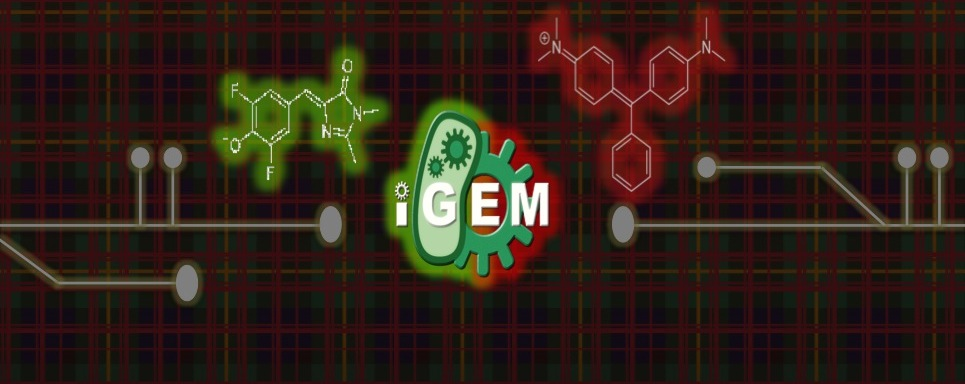# Team:Carnegie Mellon/Mod-Derivations# Documentation Preface

The documentation of the model consists of the derivations of all the equations used to create the model. Each equation contributes a piece of the picture which ultimately results in the calculations of important cell characteristics. These equations live in the Matlab model that can be found here. The characteristics we are measuring include transcriptional strength, Ts \eqref{eq:eR}, translational efficiency, Tl \eqref{eq:Tl}, and Polymerase Per Second, PoPS \eqref{eq:PoPS}.

Note: We derived equations for the model to fit the data that we obtained experimentally, while the Matlab code has even broader application and can be applied to several different experimental setups (e.g., measurement of fluorescence of both RNA and protein in the presence of degradation only, or both synthesis and degradation). These equations formed the foundation that helped extract some important cellular characteristics from the raw data that we took.

# Experimental Data Analysis

Let fluorescent mRNA and protein concentration be represented by $[R_f]$ and $[P_f]$, respectively. They are related directly to the fluorescence level, which we will label $F_r$ and $F_p$. Thus, we can write

\begin{equation}{F_r = k_r \cdot [R_f]\cdot (S_r)}\end{equation} \begin{equation}{F_p = k_p \cdot [P_f] \cdot (S_p)}\end{equation}

where $S_r$ and $S_p$ are scaling factors for mRNA and protein, respectively, and $k_r$ and $k_p$ are constants that transform fluorescence to mRNA and protein concentrations.

In the experiment, one uses a plate reader with varying concentration of the dyes in rows and varying time measurements in columns. The following image represents this.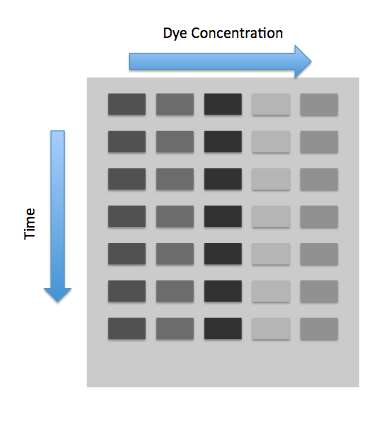We will also have another row for in vitro measurements. From this row we will graph the fluorescence versus the dye concentration, and the fluorescence will level off at some saturation point. Because the saturation point in vitro will be greater than the saturation point in vivo , we must scale all the fluorescence measurements we find in vivo , which is the importance of $S_r$ and $S_p$.

At this point we will find out the scaling factors $S_r$ and $S_p$. Step 1 is to put samples into the plate reader and take more samples of the same concentration and measure them in vitro. Then, we will measure all the wells at the same time point, and find the saturation fluorescence of the in vitro and the in vivo wells. Dividing the two gives us the $S_r$ and $S_p$.

At each time point we will graph the in vivo fluorescence vs. dye concentrations and find the first dye concentration where saturation occurs. This dye concentration is thus the mRNA/protein total concentration, as we will assume that there will be a 1-1 correspondence of dye and mRNA/protein. We then multiply each by the scaling factor $S_r$ or $S_p$ to get the actual mRNA.

# Equilibrium Constants

To check, we can find the fluorescent mRNA concentrations from the mRNA values we obtained in vivo. General first order chemical reactions begin (theoretically):

\begin{equation}\alpha [A] + \beta [B] \leftrightarrow \gamma [AB]\end{equation}

where $[AB] = 0$. So then, the equation becomes:

\begin{equation}(\alpha[A] - \gamma [AB]) + (\beta[B] - \gamma [AB]) \leftrightarrow \gamma [AB]\end{equation}

We will assume that $\alpha$, $\beta$, and $\gamma$ are all equal to 1. Our $[A]$ will be mRNA/protein and $[B]$ will be the dye concentrations. mRNA dye, which is DFHBI, will be $[D_D]$ and protein dye, which is malachite green (MG), will be $[D_M]$. Our equations are thus:

\begin{equation}([R]_{i} - [R_f]) + ([D_D] - [R_f]) \leftrightarrow ([R_f])\end{equation} \begin{equation}([P]_{i} - [P_f]) + ([D_M] - [P_f]) \leftrightarrow ([P_f])\end{equation}

The equilibrium constant $K_D$ is then defined as:

\begin{equation}K_{D_R} = \frac{[AB]}{([A]_0 - [AB]) ([B]_0 - [AB])}\end{equation}

Now inputting our variables for mRNA expression,

\begin{equation}K_{D_R} = \frac{[R_f]}{([R]_0 - [R_f])([D_R]_0 - [R_f])} \label{eq:8}\end{equation}

we can solve for $[Rf]$ using a quadratic equation based off of \eqref{eq:8}.

\begin{equation}[R_f]^2 \cdot K_{D_R} - [R_f]\cdot [K_{D_R}([R] + D_R) + 1] + K_{D_R} \cdot [R] \cdot {D_R} = 0\end{equation}

\begin{equation}[R_f] = \frac{[K_{D_R}([R][D_R]) + 1] \pm \sqrt{[K_{D_R}([R][D_R]) + 1]^2 - 4 \cdot (K_{D_R}) \cdot (K_{D_R}[R][D_R])}}{2 \cdot K_{D_R}}\end{equation}

We can apply the similar procedure for determining the protein concentration.

Degradation occurs for both mRNA and protein. After shutting off production of mRNA/protein, one can measure the degradation coefficient. Some intuition reveals that the amount that is degraded is proportional to the amount of mRNA/protein that is present:

\begin{equation}\frac{d[R]}{dt} = -\alpha \cdot [R]\end{equation}

Protein often has another constant attached to degradation, labeled maturation. Maturation $(a)$ takes into account the time it takes for a protein to mature before fluorescence can actually occur. Maturation is also dependent on the amount of protein available. In this case, the equation would be

\begin{equation}\frac{d[P]}{dt} = -(a + \beta) \cdot [P]\label{eq:12}\end{equation}

However, since the fluorogen activated protein (FAP) takes a small amount of time to fold and to bind to the dye, one can make a reasonable assumption that maturation is 0. So the simplified equation is

\begin{equation}\frac{d[P]}{dt} = -\beta \cdot [P] \label{eq:13}\end{equation}

Equations \eqref{eq:12} and \eqref{eq:13} can be solved by first order linear differential equation techniques:

\begin{equation}[R] = [R]_{max}\cdot e^{-\alpha \cdot t}\end{equation} \begin{equation}[P] = [P]_{max}\cdot e^{-\beta \cdot t}\end{equation}

From these equations $\alpha$ and $\beta$ can be determined easily.

# mRNA Expression

From the mRNA expression equations, we know that

\begin{equation}\frac{d[R]}{dt} = Ts \cdot [D] - \alpha \cdot [R]\end{equation}

where $Ts$ is the transcriptional efficiency and $\alpha$ is the degradation constant associated with mRNA degradation, and $[R]$ is the mRNA concentration or amount.

We see next that this is a first order linear equation, as $Ts$, $[D]$ and $\alpha$ are constants. Rearranging, we get

\begin{equation}\frac{d[R]}{dt} + \alpha \cdot [R] = Ts \cdot [D] \label{eq:e1}\end{equation}

The small integrating factor is thus $e^{\alpha \cdot t}$.

Multiplying the small integrating factor through equation \eqref{eq:e1} (Warning: Math ahead!)

\begin{equation}\frac{d[R]}{dt} \cdot e^{\alpha \cdot t} + \alpha \cdot [R] \cdot e^{\alpha \cdot t} = Ts \cdot [D] \cdot e^{\alpha \cdot t}\end{equation} \begin{equation}\frac{d([R]\cdot e^{\alpha \cdot t})}{dt} = Ts \cdot [D] \cdot e^{\alpha \cdot t}\end{equation} \begin{equation}[R]\cdot e^{\alpha \cdot t} = \int \! Ts \cdot [D] \cdot e^{\alpha \cdot t} \ dt\end{equation} \begin{equation}[R]\cdot e^{\alpha \cdot t} = \frac{Ts \cdot [D]}{\alpha} \cdot e^{\alpha \cdot t} + C \label{eq:e2}\end{equation}

At $t = 0$, $[R] = 0$. Plugging into \eqref{eq:e2}, we obtain:

\begin{equation}C = \frac{-Ts \cdot [D]}{\alpha}\end{equation} \begin{equation}[R] \cdot e^{\alpha \cdot t} = \frac{Ts \cdot [D]}{\alpha} \cdot e^{\alpha \cdot t} - \frac{Ts \cdot [D]}{\alpha}\end{equation} \begin{equation}[R] = \frac{Ts \cdot [D]}{\alpha} - \frac{Ts \cdot [D]}{\alpha} \cdot e^{-\alpha \cdot t}\end{equation} \begin{equation}[R] = \frac{Ts \cdot [D]}{\alpha} \cdot (1 - e^{-\alpha \cdot t})\label{eq:eR}\end{equation}

$Ts$ is then calculated by

\begin{equation} Ts = \frac{[R] \cdot \alpha}{[D] \cdot (1 - e^{-\alpha \cdot t})}\end{equation}

# Protein Expression

The protein model is a bit different from the mRNA model due to the fact that the amount of protein depends on the amount of mRNA, which is variable. mRNA is only dependent on $[D]$, which is invariable.

The basic equation looks like:

\begin{equation}\frac{d[P]}{dt} = [R] \cdot Tl - \beta \cdot [P]\end{equation}

where $[P]$ is the protein concentration or amount, $[R]$ is still mRNA, $Tl$ is the translational efficiency, and $\beta$ is the degradation constant associated with the protein.

Conveniently, we have already solved for our only hurdle to a first order linear equation, the mRNA amount (from equation \eqref{eq:eR}). We will substitute in for mRNA now:

\begin{equation}\frac{d[P]}{dt} = (1 - e^{-\alpha \cdot t}) \cdot \frac{Ts \cdot [D]}{\alpha} \cdot Tl - \beta \cdot [P]\end{equation}

Now we can solve the first order linear equation:

\begin{equation}\frac{d[P]}{dt} + \beta \cdot [P] = (1 - e^{-\alpha \cdot t}) \cdot \frac{Ts \cdot [D]}{\alpha} \cdot Tl\end{equation}

It can be seen that the integrating factor is $e^{\beta \cdot t}$ :

\begin{equation}\frac{d[P]}{dt} \cdot e^{\beta \cdot t} + \beta \cdot [P] \cdot e^{\beta \cdot t} = e^{\beta \cdot t} \cdot (1 - e^{-\alpha \cdot t}) \cdot \frac{Ts \cdot [D]}{\alpha} \cdot Tl\end{equation} \begin{equation}\frac{d([P] \cdot e^{\beta \cdot t})}{dt} = e^{\beta \cdot t} \cdot (1 - e^{-\alpha \cdot t}) \cdot \frac{Ts \cdot [D]}{\alpha} \cdot Tl\end{equation} \begin{equation}[P]\cdot e^{\beta \cdot t} = \int \! (1 - e^{-\alpha \cdot t}) \cdot \frac{Ts \cdot [D]}{\alpha} \cdot e^{\beta \cdot t} \cdot Tl\ dt\end{equation} \begin{equation}[P]\cdot e^{\beta \cdot t} = Tl \cdot \int \! \frac{Ts \cdot [D]}{\alpha} \cdot e^{\beta \cdot t} \ dt - Tl \cdot \int \!\frac{Ts \cdot [D]}{\alpha} \cdot e^{(-\alpha + \beta) \cdot t} \ dt\end{equation} \begin{equation}[P] \cdot e^{\beta \cdot t} = Tl \cdot \frac{Ts \cdot [D]}{\alpha \cdot \beta} \cdot e^{\beta \cdot t} - Tl \cdot \frac{Ts \cdot [D]}{\alpha \cdot (-\alpha + \beta)} \cdot e^{(-\alpha + \beta) \cdot t} + C \label{eq:34}\end{equation}

Now we solve for C. When $t = 0$, $P = 0$ :

\begin{equation}C = -Tl \cdot \frac{Ts \cdot [D]}{\alpha} \cdot (\frac{1}{\beta} - \frac{1}{-\alpha + \beta})\end{equation}

Substituting into \eqref{eq:34}, we obtain: \begin{equation}[P] \cdot e^{\beta \cdot t} = Tl \cdot \frac{Ts \cdot [D]}{\alpha \cdot \beta} \cdot e^{\beta \cdot t} - Tl \cdot \frac{Ts \cdot [D]}{\alpha \cdot (-\alpha + \beta)} \cdot e^{(-\alpha + \beta) \cdot t} - Tl \cdot \frac{Ts \cdot [D]}{\alpha} \cdot (\frac{1}{\beta} - \frac{1}{-\alpha + \beta}) \end{equation}

FInally, we solve for Tl. Tl is the translational efficiency, which is the second characteristic we were trying to solve for:

\begin{equation}Tl = \frac{[P]}{\frac{Ts \cdot [D]}{(\alpha \cdot \beta)} \cdot (1 - e^{-\beta \cdot t}) - \frac{Ts \cdot [D]}{\alpha \cdot (-\alpha + \beta)} \cdot (e^{-\alpha \cdot t} - e^{-\beta \cdot t})} \label{eq:Tl}\end{equation}

The following figures show that our model described above, and the parameters that we obtained fit well the measured fluorescence for the wild type (WT) promoter and three new promoters (Mutants 1,2, and 3).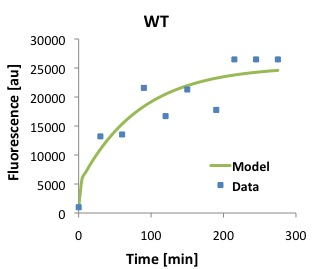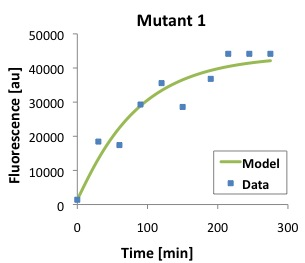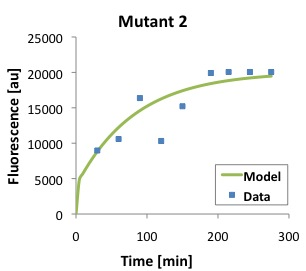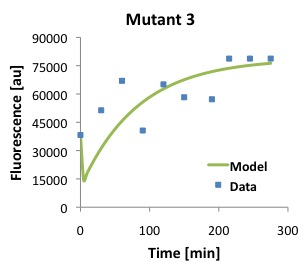# Polymerase Per Second

Taking inspiration from ”Measuring the activity of BioBrick promoters using an in vivo reference standard” by Kelly et al., we can derive our own equation for polymerase per second (PoPS), as follows.

mRNA is produced by the number of promoters times the rate of initiations of polymerase onto the promoters, or $n \cdot PoPS$. mRNA is degraded by the degradation equation we derived earlier, which is $-\alpha \cdot [R]$ :

\begin{equation}\frac{d[R]}{dt} = n \cdot PoPS - \alpha \cdot [R] \label{eq:Po1}\end{equation}

where $n$ is the number of promoters in a cell, PoPS is the rate of initiations of RNA polymerase onto the promoters.

Protein is produced by the translational efficiency times the mRNA, which is $[R] \cdot Tl$. Protein is degraded by the degradation equation we derived above, which is $-\beta \cdot [P]$ :

\begin{equation}\frac{d[P]}{dt} = [R] \cdot Tl - \beta \cdot [P] \label{eq:Po2}\end{equation}

At steady state, it can be assumed that $d[R] = 0$ and $d[P] = 0$.

So simplifying \eqref{eq:Po1} and \eqref{eq:Po2}, we obtain:

\begin{equation}PoPS = \frac{\alpha \cdot [R]}{n}\end{equation}

Substituting leaves:

\begin{equation}PoPS = \frac{\alpha \cdot \beta \cdot [P]}{n \cdot Tl} \label{eq:PoPS}\end{equation}

The output of the model is polymerase per second, which is what we have found here. It is important to realize that the purpose of finding polymerase per second is that for the current environment of a promoter and the specific type of promoter, it can be characterized using polymerase per second. Experiments can thus easily be conceived by running two experiments on the same promoter under different conditions to see how a promoter is affected, or by running two experiments on different promoters under the same conditions to see which is a stronger promoter.

 Kelly, Jason R., Adam J. Rubin, Joseph H. Davis, Caroline M. Ajo-Franklin, John Cumbers, Michael J. Czar, Kim De Mora, Aaron L. Glieberman, Dileep D. Monie, and Drew Endy. "Measuring the Activity of BioBrick Promoters Using an in Vivo Reference Standard." Journal of Biological Engineering 3.1 (2009): 4. Print.